### Measurement - Worksheets

CBSE Worksheet-01

Class - IV Mathematics – Measurement

Time Allowed: 30 minutes

1. Fill in the blanks with suitable words:
1. If the wire of 16 meters is cut into 4 pieces of the same size then the length of each piece is _____.
2. 27 kg 400 grams = ________ grams.
3. 750 ml of petrol is ____ 500 ml ____100 ml and ____ 50 ml
4. 2 kg tomatoes can be weighed by using ______ 100 g weights.
5. What should you use liters or milliliters to measure these quantities? Cough syrup in a bottle ______.
2. State whether each of the following statement is True or False:
1. There are 10 centimeter in 1 meter.
2. Centimeters, millimeters and kilometers are related with length.
3. 500 gram + 500 gram = 1kg.
4. We use the metric measure of a kilometer to measure the weight.
5. Litter, Milliliter and Kilometer are related to capacity.
3. An aeroplane is flying at a speed of 785 km per hour. How much distance will it cover in 16 hours?
1. 12500 km
2. 12560 km
3. 12650 km
4. 13560 km
4. Chandu's father is called the 'young old man' in his village. At 70 years of age, he is fully fit. Do you know his secret? He goes for a walk around the field every morning. Every day he takes four rounds of Chandu's field. What is the total distance he covers?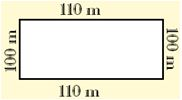1. 1806 m
2. 1680 m
3. 1860 m
4. 1608 m
5. A book shop sold 150 books on Monday. It sold 5 times more books on Tuesday than on Monday. How many books did the book shop sell on both days?
1. 750 books
2. 600 books
3. 900 books
4. 1500 books
6. How much water does a tank with equal dimensions of 3.3 m holds?
1. 35.987 cubic m
2. 35.937 cubic m
3. 35.997 cubic m
4. 35.097 cubic m
7. Amit travels 8 km 60 m by bus and 2 km 750 m by auto to reach his school from his home. How for is his school from his home?
1. 10km 810 m
2. 10 km 700 m
3. 16 km 800 m
4. 11 km 809 m
8. Match the following:

 (a) kilogram (i) mg (b) gram (ii) kg (c) milligram (iii) g (d) centigram (iv) cg
9. A tailor uses 5 m 20 cm of cloth for a suit. How much cloth will be required for 18 such suits?

10. A cloth merchant sold 18 m 60 cm cloth of type A and 44 m 90 cm type B cloth. How much total cloth did he sell?

1. 18 kg 700 g and 7 kg 350 g
2. 91 kg 350 g and 31 kg 150 g
3. 42 kg 829 g,71 kg 503 g,12 kg 391 g
4. 54 kg 378 g,63 kg 300 g, 98 kg 515 g
12. Ravi loss 5 kg 700 g weight. What was his weight if he weighs 75 kg 800 g now?

13. Convert into centimeter.

1. 35 m 99 cm
2. $\frac{1}{5}$ m
14. Convert into grams: $\frac{7}{10}$kg, $\frac{1}{6}$kg, $\frac{3}{5}$kg, $\frac{3}{4}$kg

15. Divide and find the Quotient:

1. 24 km 96 m by 12
2. 46 m 75 cm by 5
3. 18 km 126 m by 6
4. 128 m 4 cm by 2
16. Divide the following:

1. 24 km 252 m by 6
2. 18 km 360 m by 3

CBSE Worksheet-01
Class - IV Mathematics – Measurement

Solution

1. Fill in the blanks with suitable words:

1. 4 m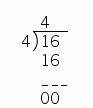16 m wire cuts in 4 equal pieces of 4 m

2. 27400
27 kg 400 g = 27 kg + 400 g
$\therefore$ 1 kg = 1000 g $⇒$ 27 kg = 27000 g
Now,
27 kg 400 g = 27000g + 400 g = 27400 gram

3. One, two, and one.
We need,
$×$ 500 = 500 ml
$×$ 100 = 200 ml
$×$ 50 = 50 ml
= 500 + 200 + 50 = 750 ml

4. Twenty
1 kg = 1000 g $⇒$ 2 kg = (2$×$1000) g = 2000 g
now,
number of 100 g weights = $\frac{2000}{100}$ = 20
2 kg tomatoes can be weighed by using twenty 100 g weights.

5. Milliliters. Cough syrup in a bottle measure in milliliters because of its capacity.

2. State whether each of the following statement is True or False:

1. False, there are 100 centimeters in 1 meter.

2. True, Centimeters, Millimeters, and Kilometers are units of length.

3. True, 500 gram + 500 gram =  1000 gram, and we know that 1kg = 1000 gram

4. False, We use the metric measure kilogram to measure the weight, while kilometer is used for length measurement.

5. False, Litter and Milliliter are related to capacity, but Kilometer is related to length.

3. (b) 12560 km
Explanation: An aeroplane is flying at a speed of 785 km per hour.
Distance will cove in 16 hours = 785 × 16 = 12560 km
4. (b) 1680 m
Explanation: Distance he covers at one round = 110 + 110 + 100 + 100 = 420 m
The total distance he covers = 4 $×$ 420 = 1680 m
5. (c) 900 books
Explanation: A book shop sold 150 books on Monday.
It sold 5 times more books on Tuesday than on Monday.
Number of books sold in Tuesday = 150 × 5 = 750 books
Total sell on both days = 750 + 150 = 900 books
6. (b) 35.937 cubic m
Explanation: Capacity of tank means volume of tank.
Volume of tank = Side × side × side = 3.3 × 3.3 × 3.3 = 35.937 cubic m
35.937 cubic m water does a tank was holds.
7. (a) 10km 810 m
Explanation: Amit travels 8 km 60 m by bus and 2 km 750 m by auto to reach his school from his home.
Distance between Amit school from his home = 8 km 60 m +  2 km 750 = 8060 + 2750 = 10810 m = 10km 810 m
8. (a) – (ii), (b) – (iii), (c) – (i), (d) – (iv)

9. Cloth use for one suit = 5 m 20 cm
As we know, 1 m = 100 cm
Then, cloth need for 18 suits = 5.20 $×$ 18 = 93.60
So, the total cloth required for 18 suits is 96 m and 60 cm

10. A cloth merchant sold 18 m 60 cm cloth of type A and 44 m 90 cm type B cloth.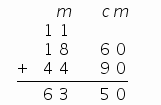Total cloth merchant sold is 63 m 50 cm

1. 18 kg 700 g and 7 kg 350 g
18.700 + 7.350 = 26 kg and 50 g
2. 91 kg 350 g and 31 kg 150 g
91.350 + 31.150 = 122 kg and 500 g
3. 42 kg 829 g, 71 kg 503 g, 12 kg 391 g
42.829 + 71.503 + 12.391 = 126 kg and 723 g
4. 54 kg 378 g, 63 kg 300 g, 98 kg 515 g
54.378 + 63.300 + 98.515 = 216 kg and 193 g
11. Ravi lost 5 kg 700 g weight, and his present weight is 75 kg 800 g.
His original weight = 5 kg 700 g + 75 kg 800 g.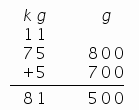81 kg 500 before he lost 5 kg 700 g weight.

12. 1 m = 100 cm

1. 35 m 99 cm
35 m 99 cm  = 35 m + 99 cm = 3500 cm + 99 cm = 3599 cm
2. $\frac{1}{5}$ m
$\frac{1}{5}$ m = $\frac{1}{5}×100=20cm$
1. $\frac{7}{10}$kg
To convert kg into gram, multiply it by 1000
$\frac{7}{10}$ $×$ 1000 = $\frac{7×1000}{10}$ = $\frac{7000}{10}$ = 700 grams
2. $\frac{1}{6}$kg
To convert kg into gram, multiply it by 1000
$\frac{1}{8}$ $×$ 1000 = $\frac{1×1000}{8}$ = $\frac{1000}{8}$ = 125 grams
3. $\frac{3}{5}$kg
To convert kg into gram, multiply it by 1000
$\frac{3}{5}$ $×$ 1000 = $\frac{3×1000}{5}$ = $\frac{3000}{5}$ = 600 grams
4. $\frac{3}{4}$kg
To convert kg into gram, multiply it by 1000
$\frac{3}{4}$ $×$ 1000 = $\frac{3×1000}{4}$ = $\frac{3000}{4}$ = 750 grams
1. 24 km 96 m by 12
We know that, 1 km = 1000 m
Then, 24.96 $÷$ 12 = 2.08
So, the quotient is 2.08
2. 46 m 75 cm by 5
We know that, 1 m = 100 cm
Then, 46.75 $÷$ 5 = 9.35
So, the quotient is 9.35
3. 18 km 126 m by 6
We know that, 1 km = 1000 m
Then, 18.126 $÷$ 6 = 3.021
So, the quotient is 3.021
4. 128 m 4 cm by 2
We know that, 1 = 100 cm
Then, 128.04 $÷$ 2 = 64.02
So, the quotient is 64.02
1. 24 km 252 m by 6
24 km 252 m = 24 km + 252 m = 24000 m + 252 m = 24252 m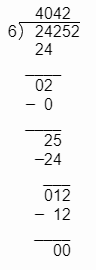$⇒$ 4 km 42 m
2. 18 km 360 m by
18 km 360 m = 18 km + 360 m = 18000 m + 360 m = 18360 m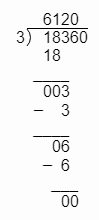$⇒$ 6 km 120 m